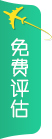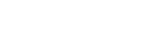• *
• *
• *

意向国家

• *

意向阶段

• *

就近城市我接受并同意 《用户服务条款》《隐私权相关政策》
【前途首页】 新东方前途出国留学，您理想的留学伙伴！
English Wibsite| 微信xdfqiantu

| 各地分公司

|关于前途分类：

43 points with 776 at HL - 39 points with 666 at HL

HL: Mathematics at 5. SL: English at 5 and Mathematics at 6 (if not at HL). Mathematical Studies is not accepted for any of our Economics degrees.

34 points with 655 at HL.

HL: Mathematics at 5. SL: English at 5 and Mathematics at 6 (if not at HL). Mathematical Studies is not accepted for any of our Economics degrees.

43 points with 776 at HL - 39 points with 666 at HL.

HL: Mathematics at 5. SL: English at 5 and Mathematics at 6 (if not at HL). Mathematical Studies is not accepted for any of our Economics degrees.

43 points with 776 at HL - 39 points with 666 at HL.

HL: Mathematics at 5. SL: English at 5 and Mathematics at 6 (if not at HL). Mathematical Studies is not accepted for any of our Economics degrees.

6,6,5 at Higher level, 35 points overall

35 points overall. 6,6,5 in Higher Level subjects

35 points overall. 6,6,5 in Higher Level subjects.

35 pointsIncluding 7,6,6 at Higher Level with HL6 in Mathematics. The total point score of 35 includes TOK/EE.

Note: IB students studying the new Maths curriculum would be required to study either Analysis and Approaches or Applications and Interpretation at Higher Level to meet the subject requirement for this programme

38 to include 666 in higher level subjects.

6,6,6 at Higher Level with a minimum of 32 points overall.

###### 英国本科经济相关专业申请要求-IB43 points with 776 at HL - 39 points with 666 at HL

HL: Mathematics at 5. SL: English at 5 and Mathematics at 6 (if not at HL). Mathematical Studies is not accepted for any of our Economics degrees.

34 points with 655 at HL.

HL: Mathematics at 5. SL: English at 5 and Mathematics at 6 (if not at HL). Mathematical Studies is not accepted for any of our Economics degrees.

43 points with 776 at HL - 39 points with 666 at HL.

HL: Mathematics at 5. SL: English at 5 and Mathematics at 6 (if not at HL). Mathematical Studies is not accepted for any of our Economics degrees.

43 points with 776 at HL - 39 points with 666 at HL.

HL: Mathematics at 5. SL: English at 5 and Mathematics at 6 (if not at HL). Mathematical Studies is not accepted for any of our Economics degrees.

6,6,5 at Higher level, 35 points overall

35 points overall. 6,6,5 in Higher Level subjects

35 points overall. 6,6,5 in Higher Level subjects.

35 pointsIncluding 7,6,6 at Higher Level with HL6 in Mathematics. The total point score of 35 includes TOK/EE.

Note: IB students studying the new Maths curriculum would be required to study either Analysis and Approaches or Applications and Interpretation at Higher Level to meet the subject requirement for this programme

38 to include 666 in higher level subjects.

6,6,6 at Higher Level with a minimum of 32 points overall.

• 您的姓名：
• 手机号码：